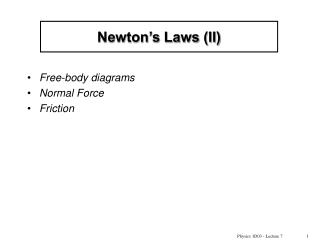# Newton’s Laws (II) - PowerPoint PPT PresentationDownload PresentationNewton’s Laws (II)

Newton’s Laws (II)
Download Presentation## Newton’s Laws (II)

- - - - - - - - - - - - - - - - - - - - - - - - - - - E N D - - - - - - - - - - - - - - - - - - - - - - - - - - -
##### Presentation Transcript

1. Newton’s Laws (II) • Free-body diagrams • Normal Force • Friction Physics 1D03 - Lecture 7

2. Free-Body Diagrams • Pick one object (the “body”). • Draw all external forces which act directly on that body (gravity, contact, electromagnetic). Imagine cutting around the body to separate it from its surroundings. Replace each external object with a force applied at the point of contact. • Indicate the direction of the acceleration of the object beside the diagram; but remember, ma is nota forceon the diagram. Physics 1D03 - Lecture 7

3. Example of a free-body diagram m A block is pulled up a frictionless ramp: • Note : • •title, to indicate the chosen object (use m or mA etc) • •contact forces, to replace the rope and the ramp • • gravity doesn’t require contact • a may be indicated for reference, but is not a force Forces on Block Physics 1D03 - Lecture 7

4. Ropes and Pulleys • A rope attached to something exerts a force parallel to the rope • The magnitude of the force is called the tension in the rope rope • Tension is uniform in a rope of negligible mass • The tension is not changed if the rope passes over an ideal pulley (assume frictionless and massless) • Tension has units of force (newtons) Force Physics 1D03 - Lecture 7

5. Contact Forces Example: We try to push a block across a table; the table pushes back. FA= applied force FA Divide the contact force from the table into two components: • normal force, perpendicular to the surfaces • friction , parallel to the surface Physics 1D03 - Lecture 7

6. Friction Friction is the force which resists sliding of two surfaces across each other. We distinguish between static and kinetic friction: Static Friction : - there is NO relative motion - fs prevents sliding Kinetic Friction : - the block is sliding - fk is opposite to v FA v Physics 1D03 - Lecture 7

7. Friction is complicated. A useful empirical model was presented by Charles Coulomb in 1781: • The force of static friction has a maximum value; if you push too hard, the block moves. This maximum value is proportional to the normal force the surfaces exert on each other. • Once the object is sliding, kinetic friction is approximately independent of velocity, and usually smaller than the maximum static friction force. The force of kinetic friction is also proportional to the normal force. Physics 1D03 - Lecture 7

8. Define two pure numbers (no units): s (“coefficient of static friction”) k (“coefficient of kinetic friction”) (“” is a Greek letter, pronounced “mu”) Then Coulomb’s rules are: Question : would it be correct to write these as vector equations, ? Physics 1D03 - Lecture 7

9. Copper on steel 0.53 0.36 Aluminum on aluminum 1.5 1.1 Teflon on Teflon 0.04 0.04 • Values depend on smoothness, temperature, etc. and are approximate • Usually m < 1, but not always • Usually, mk is less than ms , and never larger • The coefficients depend on the materials, but not on the surface areas, contact pressure, etc. Physics 1D03 - Lecture 7

10. F 100 N Example A block of mass 10kg is resting on a surface with a coefficient of static friction μs=0.50. What minimum force F is needed to pull the block? • 10 N • 49 N • 98 N Physics 1D03 - Lecture 7

11. Quiz/Example Each block weighs 100 N, and the coefficient of static friction between each pair of surfaces is 0.50. What minimum force F is needed to pull the lower block out? • 50 N • 100 N • 150 N 100 N F 100 N Physics 1D03 - Lecture 7

12. Equilibrium • A special case : (object doesn’t move, or moves at constant velocity) • Newton’s second law gives • This is equivalent to three independent component equations: • We can solve for 3 unknowns (or 2, in 2-D problems) The vector sum of forces acting on a body in equilibrium is zero Physics 1D03 - Lecture 7

13. Block on a ramp Determine all the forces acing on this block, without and with friction, given by μ. m θ Physics 1D03 - Lecture 7

14. Example A block is in equilibrium on a frictionless ramp. What is the tension in the rope? m f Physics 1D03 - Lecture 7

15. m 37o Quiz The block has weight mg and is in equilibrium on the ramp. If ms = 0.9, what is the frictional force? • 0.90 mg • 0.72 mg • 0.60 mg • 0.54 mg Physics 1D03 - Lecture 7